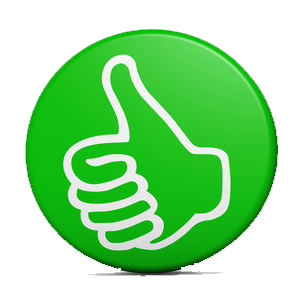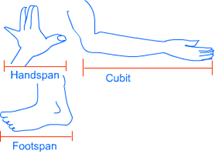### Measurement

Fun with science > Knowledge Hub > Maths > Grade 4 > Measurement

# Measurement# MEASUREMENTMEASUREMENT

Measurement has two parts; one part is the number and another part is the unit.

The known quantity which is used in measurement is called a unit.

For example: when you say that your height is 150 cm then the measurement of your height is being expressed in a number, i.e. 150 and a unit, i.e. centimeter.

Arbitrary Units of Measurement:

Before the development of standard units of measurement, people used arbitrary units of measurement.

Handspan, finger-length, cubit (length between the elbow and finger tips), foot-length and arms-length are some examples of arbitrary units of measurement.Disadvantages of Arbitrary Units of Measurement:

You have seen that arbitrary units are made up of lengths of different body parts. These lengths can vary from one person to another. This can create lot of confusion as there would be no uniformity in measurement.

STANDARD UNIT OF MEASUREMENT

To maintain uniformity in measurement, standard units of measurement were introduced in different parts of the world at different places. Napoleon is believed to have introduced most of the standard units of measurement which are being used today.

SI Unit (International System of Units):

It is the most widely used system of measurement in the world. It is based on MKS (Meter-Kilogram-Second) System.

Length, weight and time are considered as basic quantities for measurement and other quantities are derived from them.

As per SI system;

·     Unit of length is meter,

·     Unit of weight is kilogram

·     Unit of time is second.

BOOKS

We have our e-books published on Amazon for Grade 3 and Grade 4. The books serve as an important guide for Science Olympiads organized by SOF, Silverzone, Unified Council and others. Books are designed to help students understand key science concepts.

The key highlights of the book are:

·      Well explained topics

·      Use of diagrams and images for students to visualize

·      Test exercise after each chapter for self-assessment and evaluation

·      Interesting facts sections spread across the book